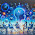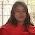## 20.5.13

### C++ Program Structure -II

The program has been structured in different lines in order to be more readable, but in C++, we do not have strict rules on how to separate instructions in different lines. For example, instead of

int main ()
{
cout << " Hello World!";
return 0;
}

We could have written:

int main () { cout << "Hello World!"; return 0; }

All in just one line and this would have had exactly the same meaning as the previous code. In C++, the separation between statements is specified with an ending semicolon (;) at the end of each one, so the separation in different code lines does not matter at all for this purpose. We can write many statements per line or write a single statement that takes many code lines. The division of code in different lines serves only to make it more legible and schematic for the humans that may read it.

Let us add an additional instruction to our first program:

// my second program in C++#include <iostream>
using namespace std;
int main ()
{
cout << "Hello World! ";
cout << "I'm a C++ program";
return 0;
}

Output:

Hello World! I'm a C++ program

In this case, we performed two insertions into cout in two different statements. Once again, the separation in
different lines of code has been done just to give greater readability to the program, since main could have been perfectly valid defined this way:

int main () { cout << " Hello World! "; cout << " I'm a C++ program "; return 0; }

We were also free to divide the code into more lines if we considered it more convenient:

int main ()
{
cout <<
"Hello World!";
cout
<< "I'm a C++ program";
return 0;
}

And the result would again have been exactly the same as in the previous examples.

Preprocessor directives (those that begin by #) are out of this general rule since they are not statements. They are lines read and processed by the preprocessor and do not produce any code by themselves. Preprocessor directives must be specified in their own line and do not have to end with a semicolon (;).

#### 8 comments:

1.id : 201420674

Write a C++ program using class to calculate square and cube of given number using inline function :

‪#‎include‬
class power
{
public:
inline int square(int n)
{
return n*n;
}
inline int cube(int n)
{
return n*n*n;
}
};
void main()
{
int n,r;
power p;
clrscr();
cout<<“\nEnter the Number: \n” ;
cin>>n;
r=p.square(n);
cout<<“\nSquare of “<<n<<” = “<<r<<endl;
r=p.cube(n);
cout<<“\nCube of “<<n<<” = “<<r<<endl;
getch();
}

2.calculator using char data input

‪#‎include‬
using namespace std;

int Add (int *x , int *y)
{
int a=*x;
int b=*y;
int c=a+b;

return (c);
}

int Sub (int *x , int *y)
{
int a=*x;
int b=*y;
int c=a-b;

return (c);
}

int Mul (int *x , int *y)
{
int a=*x;
int b=*y;
int c=a*b;

return (c);
}

int Div (int *x , int *y)
{
int a=*x;
int b=*y;
int c=a/b;

return (c);
}

int Mod (int *x , int *y)
{
int a=*x;
int b=*y;
int c=a%b;

return (c);
}

int InputFunction (int *a , int *b , char op)
{
int x=*a;
int y=*b;
int c=0;

cout<<"Please enter first number : ";
cin>>x;

cout<<"Please enter second number : ";
cin>>y;

cout<>op;

switch (op)
{
case '+':
Add (&x , &y);
break;

case '-':
Sub (&x , &y);
break;

case 'x':
Mul (&x , &y);
break;

case '/':
Div (&x , &y);
break;

case '%':
Mod (&x , &y);
break;

default:
cout<<"Your symbol is not recognized!";
break;
}

int i=c;

return (i);
}

int main()
{
int a=0;
int b=0;
char op;
char ch;
int i;

do
{
InputFunction (&a , &b , op);

int m=i;

cout<<" t t Your answer : "<>ch;

}while (ch == 'Y' || ch == 'y');

cout<<"Good- Bye"<<endl;

return 0;

}

3.id 201420674

calculator using char data input

‪#‎include‬
using namespace std;

int Add (int *x , int *y)
{
int a=*x;
int b=*y;
int c=a+b;

return (c);
}

int Sub (int *x , int *y)
{
int a=*x;
int b=*y;
int c=a-b;

return (c);
}

int Mul (int *x , int *y)
{
int a=*x;
int b=*y;
int c=a*b;

return (c);
}

int Div (int *x , int *y)
{
int a=*x;
int b=*y;
int c=a/b;

return (c);
}

int Mod (int *x , int *y)
{
int a=*x;
int b=*y;
int c=a%b;

return (c);
}

int InputFunction (int *a , int *b , char op)
{
int x=*a;
int y=*b;
int c=0;

cout<<"Please enter first number : ";
cin>>x;

cout<<"Please enter second number : ";
cin>>y;

cout<>op;

switch (op)
{
case '+':
Add (&x , &y);
break;

case '-':
Sub (&x , &y);
break;

case 'x':
Mul (&x , &y);
break;

case '/':
Div (&x , &y);
break;

case '%':
Mod (&x , &y);
break;

default:
cout<<"Your symbol is not recognized!";
break;
}

int i=c;

return (i);
}

int main()
{
int a=0;
int b=0;
char op;
char ch;
int i;

do
{
InputFunction (&a , &b , op);

int m=i;

cout<<" t t Your answer : "<>ch;

}while (ch == 'Y' || ch == 'y');

cout<<"Good- Bye"<<endl;

return 0;

}

4.id 201420674

calculator using class

‪#‎include‬

#include

using namespace std;

class calculator{

public:

calculator(){

cout << "Press 1 for addition, 2 for Subtraction, 3 for Multiplication, 4 for Division" << endl;

cin >> a;

}

void what(){

switch(a){

case 1:

cout << "Enter 2 numbers" << endl;

cin >> x >> y;

cout << endl;

cout << solution;

solution = x + y;

break;

case 2:

cout << "Enter 2 numbers" << endl;

cin >> x >> y;

cout << endl;

cout << solution;

solution = x - y;

break;

case 3:

cout << "Enter 2 numbers" << endl;

cin >> x >> y;

cout << endl;

cout << solution;

solution = x * y;

break;

case 4:

cout << "Enter 2 numbers" << endl;

cin >> x >> y;

cout << endl;

cout << solution;

solution = x / y;

break;

default:

cout << "Thank you, try again";

}

}

private:

int x;

int y;

int solution;

int a;

};

int main()

{

calculator orange;

orange.what();

return 0;

}

5.Function overloading in C++:
Md Ashraful hasan sobuj
id:201420656
B:46th
#include

class printData
{
public:
void print(int i) {
printf("Enter the value of int;
}

void print(double f) {
printf("Enter the value of float;
}

void print(char* c) {
printf("Enter the value of character;
}
};

int main(void)
{
printData pd;

// Call print to print integer
pd.print(5);
// Call print to print float
pd.print(500.263);
// Call print to print character
pd.print("Hello C++");

return 0;
}

1.Tamanna Sultana
Id: 201421041

Write a C++ program using class to calculate square and cube of given number using inline function :

#?include
class power
{
public:
inline int square(int n)
{
return n*n;
}
inline int cube(int n)
{
return n*n*n;
}
};
void main()
{
int n,r;
power p;
clrscr();
cout<<“\nEnter the Number: \n” ;
cin>>n;
r=p.square(n);
cout<<“\nSquare of “<<n<<” = “<<r<<endl;
r=p.cube(n);
cout<<“\nCube of “<<n<<” = “<<r<<endl;
getch();
}

6.Write a C++ program using class to calculate square and cube of given number using inline function :

#?include
class power
{
public:
inline int square(int n)
{
return n*n;
}
inline int cube(int n)
{
return n*n*n;
}
};
void main()
{
int n,r;
power p;
clrscr();
cout<<“\nEnter the Number: \n” ;
cin>>n;
r=p.square(n);
cout<<“\nSquare of “<<n<<” = “<<r<<endl;
r=p.cube(n);
cout<<“\nCube of “<<n<<” = “<<r<<endl;
getch();
}

7.Tamanna Sultana
Id: 201421041

Write a C++ program using class to calculate square and cube of given number using inline function :

#?include
class power
{
public:
inline int square(int n)
{
return n*n;
}
inline int cube(int n)
{
return n*n*n;
}
};
void main()
{
int n,r;
power p;
clrscr();
cout<<“\nEnter the Number: \n” ;
cin>>n;
r=p.square(n);
cout<<“\nSquare of “<<n<<” = “<<r<<endl;
r=p.cube(n);
cout<<“\nCube of “<<n<<” = “<<r<<endl;
getch();
}

Comment Here